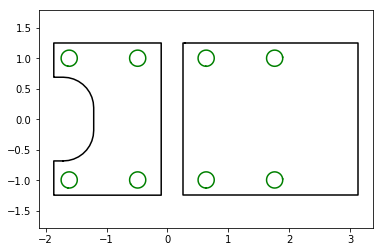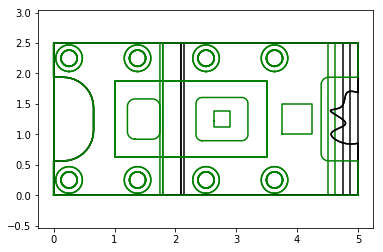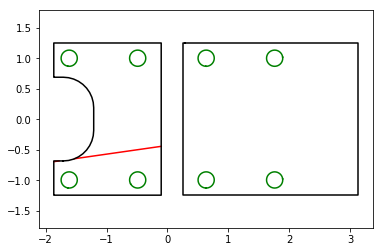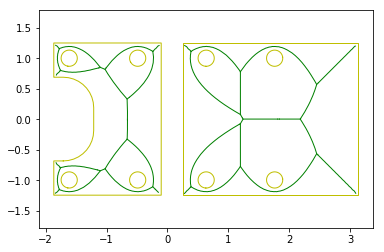In :
import trimesh
import numpy as np
from shapely.geometry import LineString
%pylab inline

Populating the interactive namespace from numpy and matplotlib

In :
# load the mesh from filename
# file objects are also supported

In :
# get a single cross section of the mesh
slice = mesh.section(plane_origin=mesh.centroid,
plane_normal=[0,0,1])

In :
# the section will be in the original mesh frame
slice.show()

Out:
In :
# we can move the 3D curve to a Path2D object easily
slice_2D, to_3D = slice.to_planar()
slice_2D.show()In :
# if we wanted to take a bunch of parallel slices, like for a 3D printer
# we can do that easily with the section_multiplane method
# we're going to slice the mesh into evenly spaced chunks along z
# this takes the (2,3) bounding box and slices it into [minz, maxz]
z_extents = mesh.bounds[:,2]
# slice every .125 model units (eg, inches)
z_levels  = np.arange(*z_extents, step=.125)

In :
# find a bunch of parallel cross sections
sections = mesh.section_multiplane(plane_origin=mesh.bounds,
plane_normal=[0,0,1],
heights=z_levels)
sections

Out:
[<trimesh.path.path.Path2D at 0x7f236086a8d0>,
<trimesh.path.path.Path2D at 0x7f2360847400>,
<trimesh.path.path.Path2D at 0x7f2358f4b240>,
<trimesh.path.path.Path2D at 0x7f2358f4b320>,
<trimesh.path.path.Path2D at 0x7f2358f4b630>,
<trimesh.path.path.Path2D at 0x7f2358f45278>,
<trimesh.path.path.Path2D at 0x7f2358f45198>,
<trimesh.path.path.Path2D at 0x7f2360873a58>,
<trimesh.path.path.Path2D at 0x7f2358fa4828>,
<trimesh.path.path.Path2D at 0x7f2358f8e908>,
<trimesh.path.path.Path2D at 0x7f2358f67208>]
In :
# summing the array of Path2D objects will put all of the curves
# into one Path2D object, which we can plot easily
combined = np.sum(sections)
combined.show()In :
# if we want to intersect a line with this 2D polygon, we can use shapely methods
polygon = slice_2D.polygons_full
# intersect line with one of the polygons
hits = polygon.intersection(LineString([[-4,-1], [3,0]]))
# check what class the intersection returned
hits.__class__

Out:
shapely.geometry.multilinestring.MultiLineString
In :
# we can plot the intersection (red) and our original geometry(black and green)
for h in hits:
plt.plot(*h.xy, color='r')
slice_2D.show()In :
# the medial axis is available for closed Path2D objects
(slice_2D + slice_2D.medial_axis()).show()In [ ]: